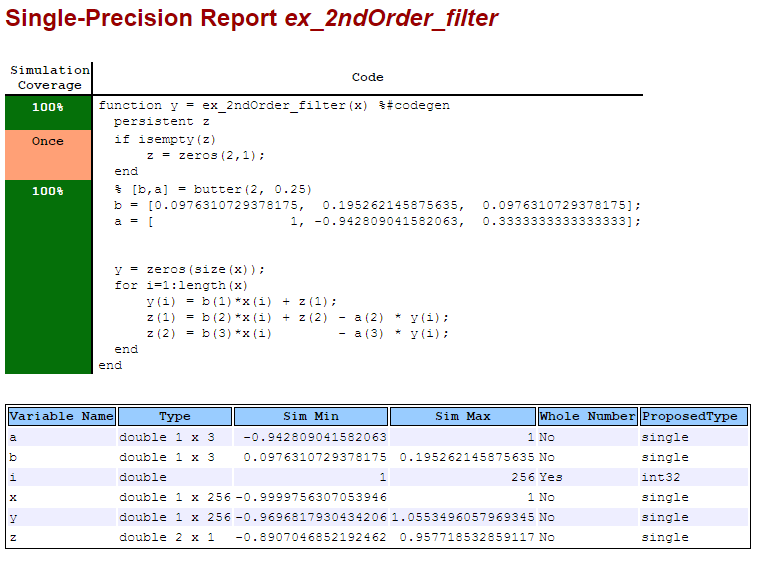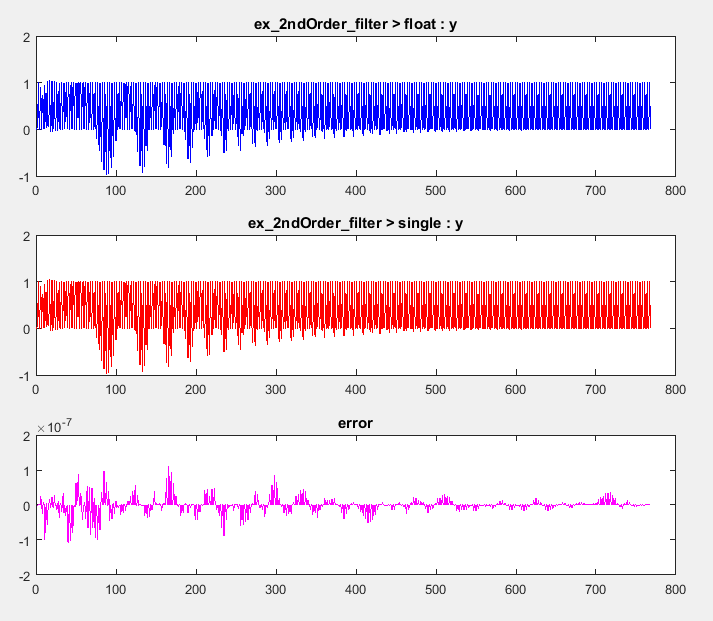Documentation

Generate Single-Precision MATLAB Code

This example shows how to generate single-precision MATLAB® code from double-precision MATLAB code.

Prerequisites

To complete this example, install the following products:

• MATLAB

• Fixed-Point Designer™

• C compiler

You can use mex -setup to change the default compiler. See Change Default Compiler (MATLAB).

Create a Folder and Copy Relevant Files

1. Create a local working folder, for example, c:\ex_2ndOrder_filter.

2. Change to the docroot\toolbox\fixpoint\examples folder. At the MATLAB command line, enter:

cd(fullfile(docroot, 'toolbox', 'fixpoint', 'examples'))

3. Copy the ex_2ndOrder_filter.m and ex_2ndOrder_filter_test.m files to your local working folder.

TypeNameDescription
Function codeex_2ndOrder_filter.mEntry-point MATLAB function
Test fileex_2ndOrder_filter_test.m

MATLAB script that tests ex_2ndOrder_filter.m

Set Up the Single-Precision Configuration Object

Create a single-precision configuration object. Specify the test file name. Verify the single-precision code using the test file. Plot the error between the double-precision code and single-precision code. Use the default values for the other properties.

scfg = coder.config('single');
scfg.TestBenchName = 'ex_2ndOrder_filter_test';
scfg.TestNumerics = true;
scfg.LogIOForComparisonPlotting = true;

Generate Single-Precision MATLAB Code

To convert the double-precision MATLAB function, ex_2ndOrder_filter, to single-precision MATLAB code, use the convertToSingle

convertToSingle -config scfg ex_2ndOrder_filter

convertToSingle analyzes the double-precision code. The conversion process infers types by running the test file because you did not specify the input types for the ex_2ndOrder_filter function. The conversion process selects single-precision types for the double-precision variables. It selects int32 for index variables. When the conversion is complete, convertToSingle generates a type proposal report.

View the Type Proposal Report

To see the types that the conversion process selected for the variables, open the type proposal report for the ex_2ndOrder_filter function. Click the link ex_2ndOrder_filter_report.html.

The report opens in a web browser. The conversion process converted:

• Double-precision variables to single.

• The index i to int32. The conversion process casts index and dimension variables to int32.View Generated Single-Precision MATLAB Code

To view the report for the generation of the single-precision MATLAB code, in the Command Window:

1. Scroll to the Generate Single-Precision Code step. Click the View report link.

2. In the MATLAB Source pane, click ex_2ndOrder_filter_single.

The code generation report displays the single-precision MATLAB code for ex_2ndOrder_filter.

View Potential Data Type Issues

When you generate single-precision code, convertTosingle enables highlighting of potential data type issues in code generation reports. If convertTosingle cannot remove a double-precision operation, the report highlights the MATLAB expression that results in the operation. Click the Code Insights tab. The absence of potential data type issues indicates that no double-precision operations remain.

Compare the Double-Precision and Single-Precision Variables

You can see the comparison plots for the input x and output y because you selected to log inputs and outputs for comparison plots .